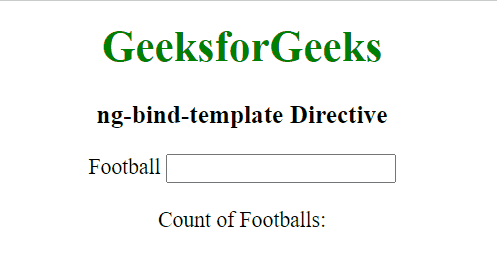# AngularJS ng-bind-template Directive

The ng-bind-template Directive in AngularJS is used to replace the content of an HTML element with the value of the given expression. It is used to bind more than one expression. It can have multiple {{ }} expressions. It is supported by all HTML elements.

Syntax: The ng-bind-template Directive can be used:

As an attribute:

```<element ng-bind-template="expression">
Contents...
</element>```

As an element:

```<ng-bind-template ng-bind-template="expression">
Content...
</ng-bind-template>```

Parameter value:

• expression: This parameter specifies more than one expression will be evaluated, where each expression is surrounded with {{}}. It is of string type.

Example 1: This example uses the ng-bind-template Directive to display the binding content.

## HTML

 `` `<``html``>`   `<``head``>` `    ``<``title``>` `        ``ng-bind-template Directive` `    ```   `    ``<``script` `src``=` `"https://ajax.googleapis.com/ajax/libs/angularjs/1.4.2/angular.min.js"``>` `    ``` ``   `<``body` `ng-app``=``"app"` `style``=``"text-align:center"``>`   `    ``<``h1` `style``=``"color:green"``>GeeksforGeeks` `    ``<``h2``>ng-bind-template Directive`   `    ``<``div` `ng-controller``=``"geek"``>` `        ``Choose one:<``br``>` `        ``<``label``>Linear Search` `            ``<``input` `type``=``"radio"` `                   ``name``=``"r1"` `                   ``ng-model``=``"search"` `                   ``value``=``"Linear Search"` `/>` `        ``<``br``>` `        ``<``label``>Binary Search` `            ``<``input` `type``=``"radio"` `                   ``name``=``"r1"` `                   ``ng-model``=``"search"` `                   ``value``=``"Binary Search"` `/>` `        ``<``br``>` `        ``<``span` `ng-bind-template``=` `            ``"{{msg}} {{search}}"``>` `        ``<``br``>` `    ```   `    ``<``script``>` `        ``var app = angular.module("app", []);` `        ``app.controller('geek', ['\$scope', ` `        ``function (\$scope) {` `            ``\$scope.msg = ` `              ``"The better searching algorithm is: ";` `            ``\$scope.search = ""` `        ``}]);` `    ``` `` ``

Output:Example 2: This example uses the ng-bind-template Directive to display the binding content.

## HTML

 `` `<``html``>`   `<``head``>` `    ``<``title``>ng-bind-template Directive`   `    ``<``script` `src``=` `"https://ajax.googleapis.com/ajax/libs/angularjs/1.4.2/angular.min.js"``>` `    ``` ``   `<``body` `ng-app``=``"app"` `style``=``"text-align:center"``>`   `    ``<``h1` `style``=``"color:green"``>GeeksforGeeks` `    ``<``h3``>ng-bind-template Directive` `    ``<``div` `ng-controller``=``"geek"``>` `        ``<``label``>Football` `            ``<``input` `min``=``"0"` `                   ``type``=``"number"` `                   ``ng-model``=``"Football"` `/>` `        ``<``br``><``br``>` `        ``Count of Footballs:` `        ``<``span` `ng-bind-template``=``"{{Football}}"``><``br``>` `    ```   `    ``<``script``>` `        ``var app = angular.module("app", []);` `        ``app.controller('geek', ['\$scope', ` `                                ``function (\$scope) {` `            ``\$scope.Football = "";` `        ``}]);` `    ``` `` ``

Output:Whether you're preparing for your first job interview or aiming to upskill in this ever-evolving tech landscape, GeeksforGeeks Courses are your key to success. We provide top-quality content at affordable prices, all geared towards accelerating your growth in a time-bound manner. Join the millions we've already empowered, and we're here to do the same for you. Don't miss out - check it out now!

Previous
Next